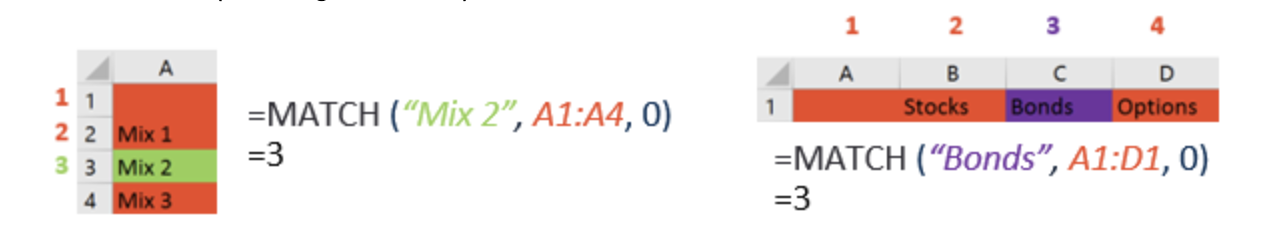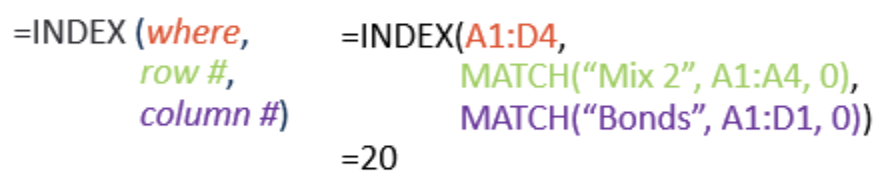# Excel Fundamentals: Lookups with INDEX-MATCH-MATCH## Topics

April 18, 2022

Last week marked the D-Lab’s inaugural “Excel Fundamentals” workshop, and to celebrate I am sharing one of my favorite Excel functions: INDEX-MATCH-MATCH. By combining the INDEX and MATCH functions, we can create a faster and more flexible lookup than the typical approach with VLOOKUP.

First, let’s explore the INDEX function and its three arguments: INDEX(where, down, across). It returns the value of a single cell within a block of data. It knows which cell we are interested in by the directions we give it: where is the range of possible cells, down is the row number within that range, and across is the column number within the same range.

In our example below, we can use INDEX to return the percentage of Portfolio Mix 2 that is allocated to bonds:Next, let’s explore the MATCH function and its three arguments: MATCH(what, where, exact?). To use MATCH, we need to tell it what to look for, where to look for it, and whether or not we need an exact match. MATCH will then return the position of the what value within the array specified in the where argument. For the purposes of this introduction, we will always assume an exact match (third argument = 0).MATCH is returning the positional arguments that we need for INDEX, so we can combine these into a single formula utilizing both INDEX and MATCH:Using INDEX-MATCH or INDEX-MATCH-MATCH provides a more flexible way to index into two-dimensional arrays. There is also evidence that it can calculate between up to 30% faster than VLOOKUP depending on the scenario, which comes in handy when dealing with large datasets and complicated workbooks. For those interested in maximizing speed and performance even beyond INDEX-MATCH-MATCH, there is a related lookup using OFFSET-MATCH-MATCH that can perform a bit faster, though many find it to be less intuitive to use. I hope that this tutorial is enough for you to consider switching your lookup-of-choice to INDEX-MATCH-MATCH!